## More on Body - Computation

A Real World Simulation is performed by solving an appropriate equation, such as the Navier-Stokes equation for fluid flow.

A Dual World Simulation is performed by solving a corresponding Dual Linearized Equations which gives information on sensitivities in the original Real World Simulation.

### Computational Simulations from the Real World

#### Movies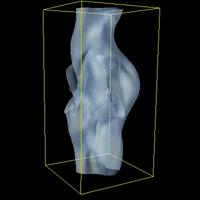Transition to turbulence in a jet flow (blowing out a candle?) (Navier-Stokes equations).
(Created by: Johan Hoffman)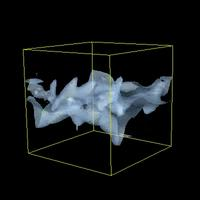Transition to turbulence in a shear flow (Navier-Stokes equations).
(Created by: Johan Hoffman)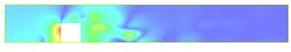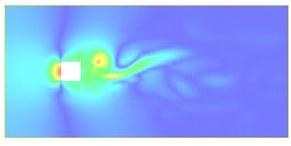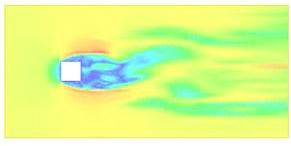Turbulent flow around a surface mounted cube at Reynolds number 40.000 (pressure in the left column, velocity in the right, viewed from the side in the top row and from above in the bottom row) (Navier-Stokes equations).
(Created by: Johan Hoffman)Channel flow with four square inlets (Navier-Stokes equations).
(Created by: Johan Hoffman)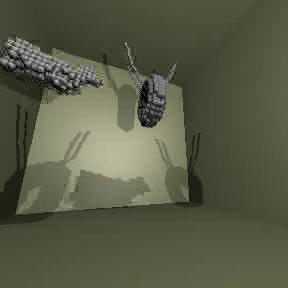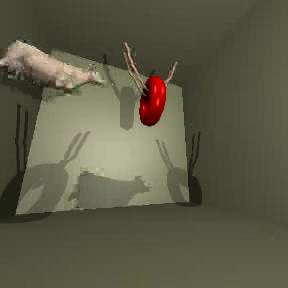Flying circus cow (physical and geometric representation) (Lagrange equations).
(Created by: Johan Jansson)

#### Still Images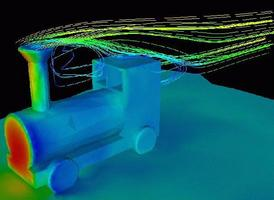Fluid flow around a train. (Navier-Stokes equations).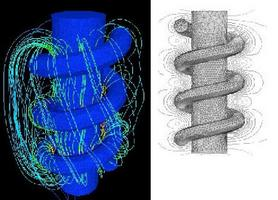Magnetic field around a coil. (Maxwell equations).

### Computational Simulations from the Dual WorldDual solution for turbulent shear flow (see above): spread of initial disturbance in the middle. (Navier-Stokes equations).
(Created by: Johan Hoffman)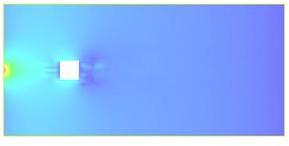Dual solution for the surface mounted cube (see above): sensitivity for computation of drag (pressure to the left, velocity to the right). (Navier-Stokes equations).
(Created by: Johan Hoffman)